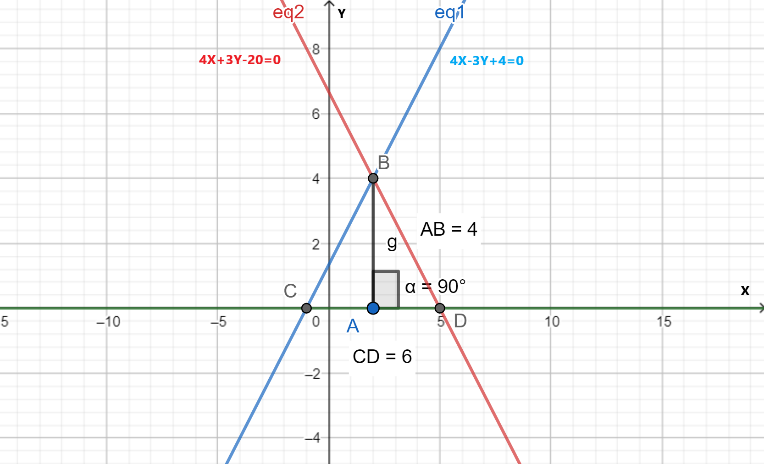QUESTION

# Solve graphically the pair of linear equations: $4x-3y+4=0$, $4x+3y-20=0$.Find the area of the region bounded by these lines and $x-axis$.(a) 12 sq. units(b) 18 sq. units(c) 9 sq. units(d) 13 sq. units

Hint: For solving this question graphically first we will find the intersection point of the given equations. Then we will plot their curve and try to find the area of the desired region.

Given:
Equations of two straight lines, $4x-3y+4=0$ and $4x+3y-20=0$ .

Let,
\begin{align} & 4x-3y+4=0.............\left( eq1 \right) \\ & 4x+3y-20=0............\left( eq2 \right) \\ \end{align}

Adding the above two equations, we get,
\begin{align} & 8x-16=0 \\ & \Rightarrow 8x=16 \\ & \Rightarrow x=2 \\ \end{align}

Now put the value of $x$ from the above equation in $eq1$ , we get,
\begin{align} & 4\times 2-3y+4=0 \\ & \Rightarrow -3y=-12 \\ & \Rightarrow y=4 \\ \end{align}

Now, we can say that the point of intersection of the given two lines will be $\left( 2,4 \right)$ .
For plotting any straight line just follow the following steps:

1. First, put x=0 in the equation and find the value of y
2. Let, for x=0 we get y=b
3. Mark point (0,b) on the y-axis
4. Similarly, put y=0 in the equation and find the value of x
5. Let, for y=0 we get x=a
6. Mark point (a,0) on the x-axis
7. Now, just the two points that we marked

Using the above steps we can plot the given straight lines. The plot of the given lines is shown below:In the above figure, $eq1$ is in blue and $eq2$ is in red.
From the plot, we can conclude that the given lines and x-axis makes a triangle DCB $\left( \Delta DCB \right)$ . And we have to find the area of this triangle.
Coordinates of the point D, C, B are:
B is the point of intersection so, coordinates of B are (2,4).
As C is the point of intersection of $eq1$ and $x-axis$ so, for coordinates of C put, $y=0$ in $eq1$ . Then,
\begin{align} & 4x-3y+4=0 \\ & \Rightarrow 4x-0=-4 \\ & \Rightarrow x=-1 \\ \end{align}
Thus, the coordinates of C are (-1,0).
Similarly, as D is the point of intersection of $eq2$ and $x-axis$ so, for coordinates of D put, $y=0$ in $eq2$ . Then,
\begin{align} & 4x+3y-20=0 \\ & \Rightarrow 4x+0=20 \\ & \Rightarrow x=5 \\ \end{align}

Thus, the coordinates of D are (5,0).
Point A is the foot of the perpendicular from point B on CD. So, AB will be perpendicular to CD or we can say that AB is parallel to the y-axis. Equation of line through AB will be $x=2$ . Then,
Coordinates of A will be (2,0).
We know that, area of any triangle $=\dfrac{1}{2}\times \left( Base \right)\times \left( Height \right)$
Here, $Base=CD$ and $Height=BA$ .
To find the length of the CD and BA we will use distance formula which is mentioned below:
If there are two points, P $\left( {{x}_{1}},{{y}_{1}} \right)$ and Q $\left( {{x}_{2}},{{y}_{2}} \right)$ . Then,
$PQ=\sqrt{{{\left( {{x}_{2}}-{{x}_{1}} \right)}^{2}}+{{\left( {{y}_{2}}-{{y}_{1}} \right)}^{2}}}$
Now, use the above formula to find the length of CD and BA.
Point B (2,4) and point A (2,0).
\begin{align} & BA=\sqrt{{{\left( 2-2 \right)}^{2}}+{{\left( 4-0 \right)}^{2}}} \\ & \Rightarrow BA=\sqrt{{{4}^{2}}} \\ & \Rightarrow BA=4 \\ \end{align}
Point C (-1,0) and point D (5,0) .
\begin{align} & CD=\sqrt{{{\left( -1-5 \right)}^{2}}+{{\left( 0-0 \right)}^{2}}} \\ & \Rightarrow CD=\sqrt{{{\left( -6 \right)}^{2}}}=\sqrt{36} \\ & \Rightarrow CD=6 \\ \end{align}
Thus, $Base=6$ and $Height=4$ .
Then, the area of $\Delta DCB=\dfrac{1}{2}\times 6\times 4=12$ sq. units.
Thus, (a) is the correct option.

Note: Here, students must plot the given equations carefully and find the intersection point. For calculating the area first figure out the region for which we have to find the area according to the given constraints.